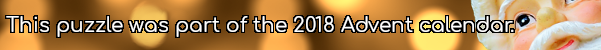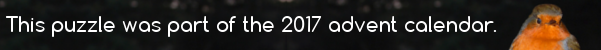mscroggs.co.uk
mscroggs.co.uksubscribe

# Puzzles

## 21 DecemberPut the digits 1 to 9 (using each digit exactly once) in the boxes so that the sums are correct. The sums should be read left to right and top to bottom ignoring the usual order of operations. For example, 4+3×2 is 14, not 10. Today's number is the smallest number you can make using the digits in the red boxes.
 + ÷ = 2 × + - × - = 31 + + - - × = 42 =37 =13 =-2
Tags: numbers, grids

## 16 DecemberArrange the digits 1-9 in a 3×3 square so that the first row makes a triangle number, the second row's digits are all even, the third row's digits are all odd; the first column makes a square number, and the second column makes a cube number. The number in the third column is today's number.
 triangle all digits even all digits odd square cube today's number
Tags: numbers, grids

## 14 DecemberPut the digits 1 to 9 (using each digit exactly once) in the boxes so that the sums are correct. The sums should be read left to right and top to bottom ignoring the usual order of operations. For example, 4+3×2 is 14, not 10. Today's number is the product of the numbers in the red boxes.
 - + = 10 ÷ + ÷ ÷ + = 3 + - ÷ + × = 33 =7 =3 =3
Tags: numbers, grids

## 8 DecemberArrange the digits 1-9 in a 3×3 square so: each digit the first row is the number of letters in the (English) name of the previous digit, each digit in the second row is one less than the previous digit, each digit in the third row is a multiple of the previous digit; the second column is an 3-digit even number, and the third column contains one even digit. The number in the first column is today's number.
 each digit is the number of letters in the previous digit each digit is one less than previous each digit is multiple of previous today's number even 1 even digit
Edit: There was a mistake in this puzzle: the original had two solutions. If you entered the wrong solution, it will automatically change to the correct one.
Tags: numbers, grids

## 3 DecemberPut the digits 1 to 9 (using each digit exactly once) in the boxes so that the sums are correct. The sums should be read left to right and top to bottom ignoring the usual order of operations. For example, 4+3×2 is 14, not 10. Today's number is the product of the numbers in the red boxes.
 + + = 11 - + × + - = 11 - - - + + = 11 =-11 =11 =11
Tags: numbers, grids

## 19 DecemberPut the digits 1 to 9 (using each digit exactly once) in the boxes so that the products are correct. Today's number is the smallest number that can be made using the digits in the red boxes.
 × × = 90 × × × × × = 84 × × × × × = 48 =64 =90 =63
Tags: numbers, grids

## 17 DecemberArrange the digits 1-9 in a 3×3 square so that every row makes a three-digit square number, the first column makes a multiple of 7 and the second column makes a multiple of 4. The number in the third column is today's number.
 square square square multiple of 7 multiple of 4 today's number
Tags: numbers, grids

## 12 DecemberPut the digits 1 to 9 (using each digit exactly once) in the boxes so that the sums are correct. Today's number is the product of the numbers in the red boxes.
 + + = 17 + + + + + = 7 + + + + + = 21 =11 =20 =14
Tags: numbers, grids

## Archive

Show me a random puzzle
▼ show ▼